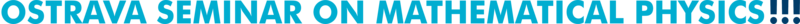Past talks (2016)
 1. February 12, 2016 Andrii Khrabustovskyi (Karlsruhe Institute of Technology) Periodic quantum graphs with predefined spectral gaps Abstract   Slides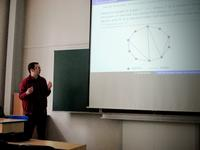2. March 22, 2016 Jiří Lipovský (University of Hradec Králové) On the effective size of a non-Weyl graph Abstract   Slides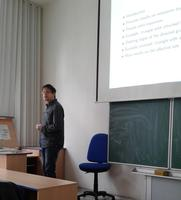3. April 4, 2016 Anton Galaev (University of Hradec Králové) Holonomy groups of Lorentzian manifolds and their applications Abstract: A short introduction to the theory of the holonomy groups for pseudo-Riemannian manifolds will be given. A survey of recent results on classification of the connected holonomy groups of Lorentzian manifolds will be presented. Some applications of this classification will be considered. Literature: A.S. Galaev, Holonomy groups of Lorentzian manifolds, Russ. Math. Surv. 70 (2015), 249-298. Slides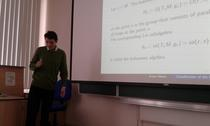4. May 10, 2016 Mariusz Hynek (KTH Royal Institute of Technology, Stockholm) Matrix models describing the quantum bosonic membrane in the large N limit Abstract: I will review the concept of matrix regularization of a smooth 2-dimensional compact manifold $$M$$, which consists in replacing the algebra of smooth functions on $$M$$ by $$SU(N)$$ matrices. Some properties of $$M$$ can then be restored by taking the limit $$N \rightarrow \infty$$. The procedure will be used to establish a quantum description of so called relativistic membranes, i.e. submanifolds of Minkowski space with vanishing mean curvature, having locally the topology $$\mathbb{R} \times M$$. The family of $$SU(N)$$ regularizations of $$M$$ allows to replace the corresponding formal, a priori ill-defined, quantum field theory with Hamiltonian $$H$$ by a sequence of self-adjoint Schrödinger operators $$H_N$$ with purely discrete spectrum, such that classically $$H = \lim_{N \rightarrow \infty} H_N$$. I will prove some bounds for the spectrum of $$H_N$$ using Fock space techniques and I will point out their applications to embedded eigenvalues in the continuous spectrum of the Supersymmetric Matrix Models. Literature: M. Hynek, Optimized Fock space in the large N limit of quartic interactions in matrix models, Nucl. Phys. B 906 (2016), 497-523; arXiv:1512.02969. Slides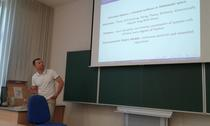5. May 11, 2016 Mariusz Hynek (KTH Royal Institute of Technology, Stockholm) Singularities of relativistic membranes Abstract: I will present the problem of singularity formation for relativistic strings and membranes. The vanishing mean curvature condition in Minkowski space generates a geometric flow for the underlying compact manifold, which turns out to be exactly solvable in the case of a string. I will review some known results and I will point out the connection with the Eikonal equation in the case of membranes, which will be used to study their singularities. I will derive a class of self-similar solutions of the flow equations around a singularity as well as I will present the results of numerical simulations showing the formation of singularities from smooth initial conditions. Literature: J. Eggers, J. Hoppe, M. Hynek, and N. Suramlishvili, Singularities of relativistic membranes, Geometric Flows 1 (2015), 17-33. Slides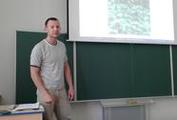6. June 2, 2016 Vladimir Lotoreichik (Nuclear Physics Institute, Czech Academy of Sciences) Leaky conical surfaces: spectral asymptotics, isoperimetric properties, and beyond Abstract   Slides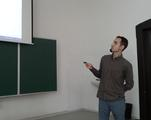7. July 7, 2016 Geoff Prince & Thoan Do (La Trobe University, Melbourne) A classification scheme for the inverse problem in arbitrary dimension Abstract: We present a new classification scheme for the inverse problem in the calculus of variations in arbitrary dimension. This generalises the Douglas scheme for a pair of second order ODEs in a surprising way showing that Douglas "got it wrong" but in dimension 2 this is immaterial. We also give a comprehensive treatment of the hard case where some eigenspaces of the Jacobi endomorphism are integrable and some are not. Slides:   Geoff Prince   Thoan Do 8. July 8, 2016 Dennis Barrett (Rhodes University, Grahamstown, South Africa) Invariant nonholonomic Riemannian structures on three-dimensional Lie groups Slides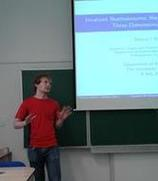9. September 27, 2016 Sylwia Kondej (University of Zielona Góra) Discrete eigenvalues asymptotics in two models with delta interaction Abstract: We discuss two two-dimensional quantum systems with interactions supported by lines in $$\mathbb R^2$$. More precisely, in the first model the potential support is determined by an angle and we analyze the spectral asymptotics if the angle-shape line approaches to the straight line. In the second model the potential support is defined by two parallel lines and the eigenvalues asymptotics is derived if both lines approach each other. Finally, some generalizations of the above mentioned systems will be discussed. The results presented in the talk are based on the common works with P. Exner and D. Krejcirik. Slides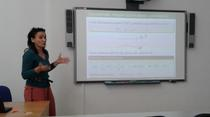10. November 22, 2016 David Saunders (University of Ostrava) Jets -- or, how to geometrize the differential calculus Abstract: The idea of a "jet" was introduced by Charles Ehresmann in 1951 as an equivalence class of maps, all defined in some neighbourhood of a given point, and with the same value and derivatives (up to a given order) at that point. Conceptually, therefore, a jet may be considered as an abstract Taylor polynomial. In this talk I shall expand on this definition, and explain the structure of various spaces of jets (of which the simplest is the tangent bundle of a differentiable manifold). I shall also explain how the use of jets can give a precise meaning to certain aspects of the Euler-Lagrange equations of the calculus of variations, such as the ideas of "differentiating with respect to derivatives" and of "total derivative". Slides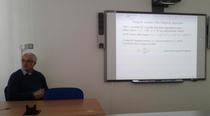11. December 13, 2016 David Krejčiřík (Nuclear Physics Institute, Czech Academy of Sciences) Spectral geometry of tubes Abstract: We shall make an overview of the interplay between the geometry of tubular neighbourhoods of Riemannian manifolds and the spectrum of the associated Dirichlet Laplacian. An emphasis will be put on the existence of curvature-induced eigenvalues in bent tubes and Hardy-type inequalities in twisted tubes of non-circular cross-section. Consequences of the results for physical systems modelled by the heat or Schroedinger equations will be also discussed. Slides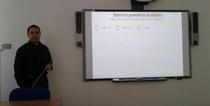12. December 13, 2016 Mirosław Lachowicz (University of Warsaw) Self-organization and multiscale approach: a mathematical theory Abstract   Slides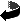Ostrava Mathematical Seminar
Created: Wed Feb 15 2017